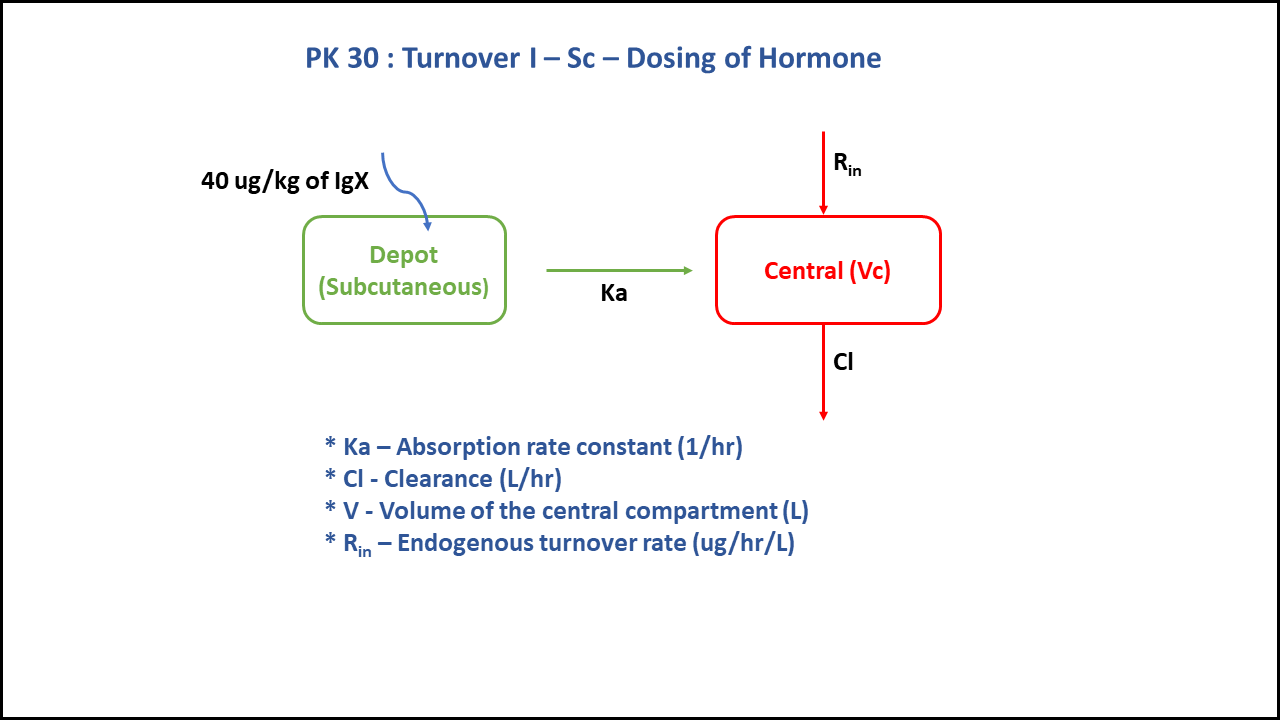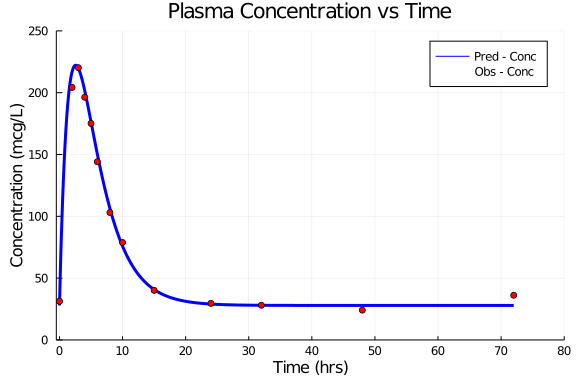# Exercise PK30 - Turnover I - SC - Dosing of hormone

### Background

• Structural model - One compartment first order elimination with zero order production of hormone

• Route of administration - Subcutaneous

• Dosage Regimen - 40 mcg/kg

• Number of Subjects - 1### Learning Outcome

Since we had subcutaneous dose information, we learnt to discriminate between the clearance and the rate of synthesis. Further simplification of the model by assuming bioavailability is 100% and concentration of the endogenous compound equals concentration at baseline (turnover/clearance)

### Objectives

In this tutorial, you will learn how to build a one compartment PK turnover model, following first order elimination kinetics and zero order hormone production.

### Libraries

call the "necessary" libraries to get started

using Pumas
using Plots
using CSV
using StatsPlots
using Random


### Model

In this two compartment model, we administer dose subcutaneously.

pk_30           = @model begin
@param begin
tvka        ∈ RealDomain(lower=0)
tvcl        ∈ RealDomain(lower=0)
tvsynthesis ∈ RealDomain(lower=0)
tvv         ∈ RealDomain(lower=0)
Ω           ∈ PDiagDomain(4)
σ_add       ∈ RealDomain(lower=0)
end

@random begin
η           ~ MvNormal(Ω)
end

@pre begin
Ka          = tvka * exp(η)
Cl          = tvcl * exp(η)
Synthesis   = tvsynthesis * exp(η)
V           = tvv * exp(η)
end

@init begin
Central     = Synthesis/(Cl/V) # Concentration at Baseline = Turnover Rate (0.78) / Cl of hormone (0.028)
end

@dynamics begin
Depot'      = -Ka * Depot
Central'    =  Ka * Depot + Synthesis - (Cl/V) * Central
end

@derived begin
cp          = @. Central/V
dv          ~ @. Normal(cp, σ_add)
end
end

PumasModel
Parameters: tvka, tvcl, tvsynthesis, tvv, Ω, σ_add
Random effects: η
Covariates:
Dynamical variables: Depot, Central
Derived: cp, dv
Observed: cp, dv


### Parameters

The parameters are as given below. tv represents the typical value for parameters.

• Ka - Absorption rate constant (hr⁻¹)

• Cl - Clearance (L/kg/hr)

• Synthesis - Turnover Rate (hr⁻¹)

• V - Volume of Central Compartment (L/kg)

• Ω - Between Subject Variability

• σ - Residual error

param =  ( tvka         = 0.539328,
tvcl         = 0.0279888,
tvsynthesis  = 0.781398,
tvv          = 0.10244,
Ω            = Diagonal([0.0,0.0,0.0,0.0]),
σ_add        = 3.97427)

(tvka = 0.539328, tvcl = 0.0279888, tvsynthesis = 0.781398, tvv = 0.10244,
Ω = [0.0 0.0 0.0 0.0; 0.0 0.0 0.0 0.0; 0.0 0.0 0.0 0.0; 0.0 0.0 0.0 0.0], σ
_add = 3.97427)


### Dosage Regimen

A dose of 40 mcg/kg is given subcutaneously to a Single subject.

ev1  = DosageRegimen(40,time=0,cmt=1)
sub1 = Subject(id=1, events=ev1)

Subject
ID: 1
Events: 1


### Simulation

To simulate plasma concentration with turnover rate after oral administration.

Random.seed!(1234)
sim_sub1 = simobs(pk_30, sub1, param, obstimes=0:0.1:72)
df1      = DataFrame(sim_sub1)


### Dataframe & Plot

Use the dataframe for plotting

df1_dv = filter(x -> x.time in [0, 2, 3, 4, 5, 6, 8, 10, 15, 24, 32, 48, 72], df1)

@df df1 plot(:time, :cp,
label= "Pred - Conc",  xlabel="Time (hrs)", ylabel="Concentration (mcg/L)",
title="Plasma Concentration vs Time", color=[:blue], linewidth=3,
xlims=(-0.5,80), xticks=[0,10,20,30,40,50,60,70,80], ylims=(0,250), yticks=[0,50,100,150,200,250])
@df df1_dv scatter!(:time, :dv, label="Obs - Conc", color=[:red])